# Broyden-Fletcher-Goldfarb-Shanno method

(diff) ← Older revision | Latest revision (diff) | Newer revision → (diff)

BFGS method

The unconstrained optimization problem is to minimize a real-valued functionof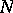variables. That is, to find a local minimizer, i.e. a point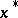such that(a1)is typically called the cost function or objective function.

A classic approach for doing this is the method of steepest descent (cf. Steepest descent, method of). The iteration, here described in terms of the transition from a current approximation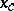to a local minimizer, to an update (and hopefully better) approximation is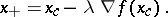(a2)

By elementary calculus,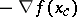is the direction of most rapid decrease (steepest descent) instarting from. The step lengthmust be a part of the algorithm in order to ensure that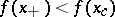(which must be so for a sufficiently small).

There are several methods for selecting an appropriate, [a3], [a7], for instance the classical Armijo rule, [a1], in whichfor some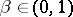and whereis the least integer such that the sufficient decrease condition(a3)

holds. In (a3),is replaced by a general descent direction, a vector satisfying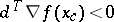, and a parameterhas been introduced (typically). One might think that simple decrease (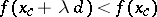) would suffice, and it usually does in practice. However, the theory requires (a3) to eliminate the possibility of stagnation.

The steepest descent iteration with the Armijo rule will, ifis smooth and bounded away from, which is assumed throughout below, produce a sequence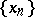such that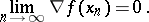(a4)

Hence, any limit pointof the steepest descent sequence satisfies the first-order necessary conditions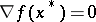. This does not imply convergence to a local minimizer and even if the steepest descent iterations do converge, and they often do, the convergence is usually very slow in the terminal phase when the iterates are near. Scaling the steepest descent direction by multiplying it with the inverse of a symmetric positive-definite scaling matrixcan preserve the convergence of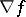to zero and dramatically improve the convergence near a minimizer.

Now, ifis a local minimizer that satisfies the standard assumptions, i.e. the Hessian of,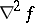, is symmetric, positive definite, and Lipschitz continuous near, then the Newton method,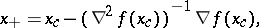(a5)

will converge rapidly to the minimizer if the initial iterate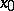is sufficiently near, [a3], [a7]. However, when far from, the Hessian need not be positive definite and cannot be used as a scaling matrix.

Quasi-Newton methods (cf. also Quasi-Newton method) update an approximation ofas the iteration progresses. In general, the transition from current approximationsandofand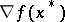to new approximations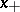andis given (using a line search paradigm) by:

1) compute a search direction;

2) find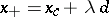using a line search to ensure sufficient decrease;

3) use,, andto update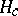and obtain. The way in which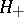is computed determines the method.

The BFGS method, [a2], [a4], [a5], [a6], updateswith the rank-two formula(a6)

In (a6),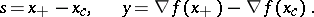## Local and global convergence theory.

The local convergence result, [a8], [a3], [a7], assumes that the standard assumptions hold and that the initial data is sufficiently good (i.e.,is near toand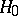is near). Under these assumptions the BFGS iterates exist and converge-superlinearly to, i.e.,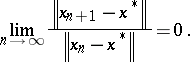(a7)

By a global convergence theory one means that one does not assume that the initial data is good and one manages the poor data by making certain thatis decreased as the iteration progresses. There are several variants of global convergence theorems for BFGS and related methods, [a9], [a11], [a10], [a12]. All these results make strong assumptions on the function and some require line search methods more complicated that the simple Armijo rule discussed above.

The result from [a9] is the most general and a special case illustrating the idea now follows. One must assume that the setis convex,is Lipschitz twice continuously differentiable in, and that the Hessian and its inverse are uniformly bounded and positive definite in. This assumption implies thathas a unique minimizerinand that the standard assumptions hold. Ifis symmetric and positive definite, the BFGS iterations, using the Armijo rule to enforce (a3), converge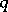-superlinearly to.

## Implementation.

The recursive implementation from [a7], described below, stores the history of the iteration and uses that information recursively to compute the action of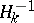on a vector. This idea was suggested in [a13], [a14], [a17]. Other implementations may be found in [a15], [a3], [a7], and [a16].

One can easily show that(a8)

The algorithm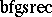below overwrites a given vectorwith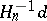. The storage needed is one vector for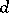and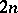vectors for the sequences. A method for computing the product of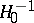and a vector must also be provided.

'

<tbody> </tbody>
 1 IF,; return 2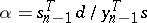;call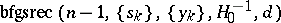; 3.
 [a1] L. Armijo, "Minimization of functions having Lipschitz-continuous first partial derivatives" Pacific J. Math. , 16 (1966) pp. 1–3 [a2] C.G. Broyden, "A new double-rank minimization algorithm" Notices Amer. Math. Soc. , 16 (1969) pp. 670 [a3] J.E. Dennis, R.B. Schnabel, "Numerical Methods for Nonlinear Equations and Unconstrained Optimization" , Classics in Applied Math. : 16 , SIAM (Soc. Industrial Applied Math.) (1996) [a4] R. Fletcher, "A new approach to variable metric methods" Comput. J. , 13 (1970) pp. 317–322 [a5] D. Goldfarb, "A family of variable metric methods derived by variational means" Math. Comp. , 24 (1970) pp. 23–26 [a6] D.F. Shanno, "Conditioning of quasi-Newton methods for function minimization" Math. Comp. , 24 (1970) pp. 647–657 [a7] C.T. Kelley, "Iterative methods for optimization" , Frontiers in Appl. Math. , 18 , SIAM (Soc. Industrial Applied Math.) (1999) [a8] C.G. Broyden, J.E. Dennis, J.J. Moré, "On the local and superlinear convergence of quasi-Newton methods" J. Inst. Math. Appl. , 12 (1973) pp. 223–246 [a9] R.H. Byrd, J. Nocedal, "A tool for the analysis of quasi-Newton methods with application to unconstrained minimization" SIAM J. Numer. Anal. , 26 (1989) pp. 727–739 [a10] R.H. Byrd, J. Nocedal, Y. Yuan, "Global convergence of a class of quasi-Newton methods on convex problems" SIAM J. Numer. Anal. , 24 (1987) pp. 1171–1190 [a11] M.J.D. Powell, "Some global convergence properties of a variable metric algorithm without exact line searches" R. Cottle (ed.) C. Lemke (ed.) , Nonlinear Programming , Amer. Math. Soc. (1976) pp. 53–72 [a12] J. Werner, "Über die globale konvergenz von Variable-Metric Verfahren mit nichtexakter Schrittweitenbestimmung" Numer. Math. , 31 (1978) pp. 321–334 [a13] K.J. Bathe, A.P. Cimento, "Some practical procedures for the solution of nonlinear finite element equations" Comput. Meth. Appl. Mech. Eng. , 22 (1980) pp. 59–85 [a14] H. Matthies, G. Strang, "The solution of nonlinear finite element equations" Internat. J. Numerical Methods Eng. , 14 (1979) pp. 1613–1626 [a15] R.H. Byrd, J. Nocedal, R.B. Schnabel, "Representation of quasi-Newton matrices and their use in limited memory methods" Math. Progr. , 63 (1994) pp. 129–156 [a16] J.L. Nazareth, "Conjugate gradient methods less dependent on conjugacy" SIAM Review , 28 (1986) pp. 501–512 [a17] J. Nocedal, "Updating quasi-Newton matrices with limited storage" Math. Comp. , 35 (1980) pp. 773–782
How to Cite This Entry:
Broyden-Fletcher-Goldfarb-Shanno method. Encyclopedia of Mathematics. URL: http://encyclopediaofmath.org/index.php?title=Broyden-Fletcher-Goldfarb-Shanno_method&oldid=17420
This article was adapted from an original article by C.T. Kelley (originator), which appeared in Encyclopedia of Mathematics - ISBN 1402006098. See original article